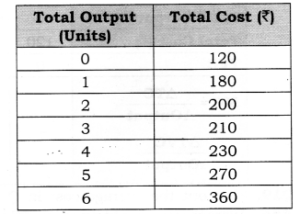# Chapter 6 – Cost Questions and Answers: NCERT Solutions for Class 12 Micro Economics

Class 12 Introduction to Economics NCERT book solutions for Chapter 6 - Cost Questions and Answers.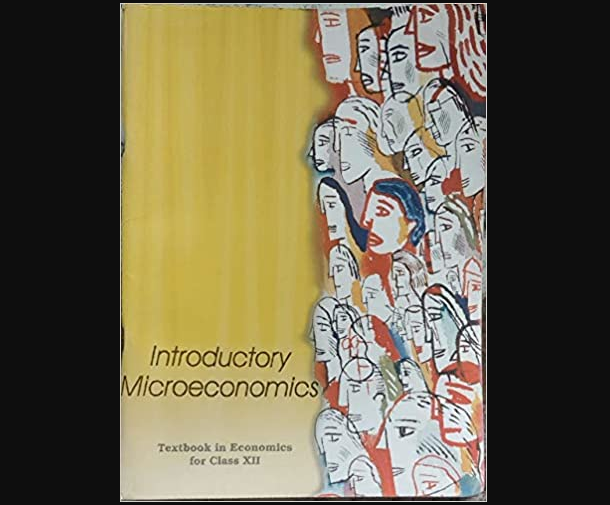## Question 2. What are total fixed cost, total variable cost and total cost of a firm? How are they related? Or Draw TVC, TC, and TFC curves in a single diagram.

### Answer: (i) TC is divided into two parts TFC and TVC such that TC = TFC + TVC. (ii) TFC is the overhead cost and it remains constant or fixed whatever be the level of output. TFC curve is a horizontal line parallel to the x-axis. (iii) TVC is cost due to increased use of variable factors like raw material, labour, etc. TVC is inverse S-shaped starting from the origin due to law of variable proportion. (iv) TC is aggregate of TFC and TVC. TC curve is inverse S-shaped starting from the level of fixed cost. The reason behind it shape is the law of variable proportion.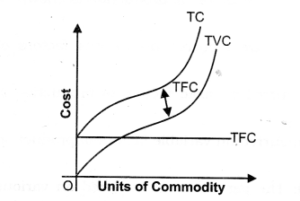## Question 9. Why is the short run marginal cost curve U- Shaped?

### Answer: Marginal cost is U-shaped because of Law of variable proportion: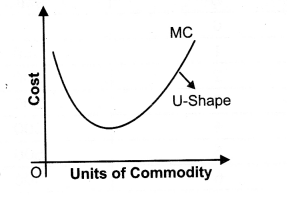## Question 11. The following table shows the total cost schedule of a firm. What is the total fixed cost schedule of this firm? Calculate the TVC, AFC, AVC, SAC and SMC schedules of the firm.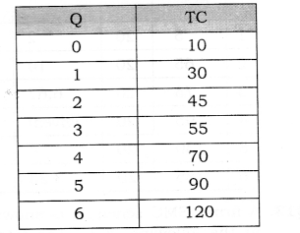## Question 12. The following table gives the total cost schedule of a firm. It Is also given that the average fixed cost at 4 units of output is Rs. 5.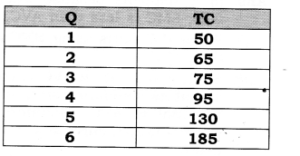## Find the TVC, TFC. AVC, AFC, SAC and SMC schedules of the firm for the corresponding values of output.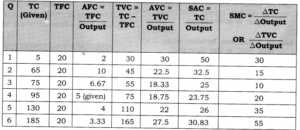## Question 13. A firm’s SMC schedule is shown in the following table. The total fixed cost of the firm is Rs. 100.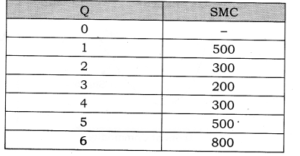## Find the TVC, TC, AVC and SAC schedules of the firm.[6 Marks]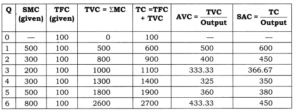## Question 3. Explain the behaviour of aver age fixed cost using numerical example.

### Answer: (i) The per unit cost incurred on fixed factors of production is known as average fixed cost.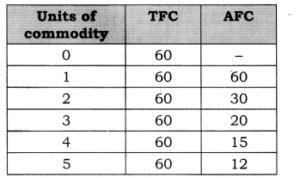## Question 4. Distinguish between variable cost and fixed cost. Give two examples of each.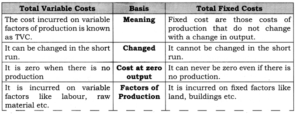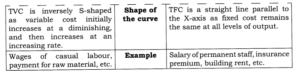## Question 5. Why is AC curve U-shaped in short run? Or Why is AC curve U-shaped?

### Answer: Average Cost is U-Shaped because of Law of variable proportion: (i) The shape of average cost (AC) depends upon total cost (TC). (ii) Initially, total cost (TC) increases at a diminishing rate (Total Product increases at Increasing rate), which makes its average, i.e., average cost (AC) to fall, then reaches its minimum point.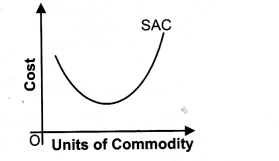## Question 7. State the distinction between explicit each.Give an example of each?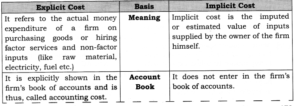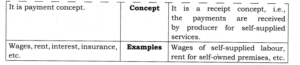## Question 11. Define cost. State the relation between marginal cost and average variable cost.

### Answer: Cost is the sum total of explicit cost, implicit cost and certain minimum profit (normal profit). (i) As long as MC is below AVC, AVC curve falls till their intersection at point E. (ii) When MC curve comes to fall, it falls more rapidly than AVC curve and reaches its minimum point B earlier than the AVC curve reaches its minimum point E. Therefore, MC curve is rising from B to E whereas AVC curve is still falling from A to E. (iii) When MC curve is rising, it cuts the AVC curve at its minimum point E and after that point MC is above than AVC.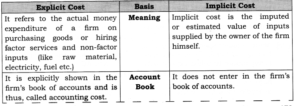## Question 14. Find out the total fixed cost in the following: